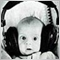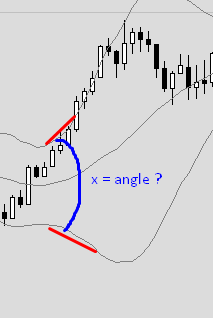Cant find and use trendline angle - page 2240

I am sorry to bring this post up, I just couldn't find the answer here and it bothered me to see answers like "it can't be done" etc.

So, it CAN be done, using my example below.

First I will explain what needs to be done, since we all agree that the angle cannot be determined if your coordinates are (price,time), so :

1. First challenge is that you need to change them to the chart's (x,y), so we'll get what we see visually.

2. Second challenge is that (x,y) are also not on the same scale, so you need to multiply your resulted slope by the width/height ratio.

So the code should be something like:

```#define PI           3.14159265359

double GetAngle(datetime time1,double price1,datetime time2,double price2)
{
int x1,y1,x2,y2;

// Convert the (time,price) coordinates to (x,y) coordinates
ChartTimePriceToXY(0,0,time1,price1,x1,y1);
ChartTimePriceToXY(0,0,time2,price2,x2,y2);

// Flip Y coordinates because screen point (0,0) is upper-leftmost
//   and not lower-leftmost as we usually draw the axes on paper
y1=ChartHeightInPixelsGet()-y1;
y2=ChartHeightInPixelsGet()-y2;

//Calculate the slope, notice the forced casting that need to be done
double m=(double)(y2-y1)/(double)(x2-x1)*((double)ChartWidthInPixels()/(double)ChartHeightInPixelsGet());

//Calculate the angle in degrees

return(angle);
}
//+------------------------------------------------------------------+
//| The function receives the chart width in pixels.                 |
//+------------------------------------------------------------------+
int ChartWidthInPixels(const long chart_ID=0)
{
//--- prepare the variable to get the property value
long result=-1;
//--- reset the error value
ResetLastError();
if(!ChartGetInteger(chart_ID,CHART_WIDTH_IN_PIXELS,0,result))
{
//--- display the error message in Experts journal
Print(__FUNCTION__+", Error Code = ",GetLastError());
}
//--- return the value of the chart property
return((int)result);
}

//+------------------------------------------------------------------+
//| The function receives the chart height value in pixels.          |
//+------------------------------------------------------------------+
int ChartHeightInPixelsGet(const long chart_ID=0,const int sub_window=0)
{
//--- prepare the variable to get the property value
long result=-1;
//--- reset the error value
ResetLastError();
if(!ChartGetInteger(chart_ID,CHART_HEIGHT_IN_PIXELS,sub_window,result))
{
//--- display the error message in Experts journal
Print(__FUNCTION__+", Error Code = ",GetLastError());
}
//--- return the value of the chart property
return((int)result);
} ```628

fridayda13:

1. First challenge is that you need to change them to the chart's (x,y), so we'll get what we see visually.

2. Second challenge is that (x,y) are also not on the same scale, so you need to multiply your resulted slope by the width/height ratio.

1. is necessary

2 . (x,y)  are in pixel coordinates system so they are in  same scale : 1 pixel in x-direction is equal to 1 pixel in y-direction. So no need to get a ratio

--------------------------

Another point : no also need  "to flip Y coord. from upper-leftmost to lower-leftmost"240

paulselvan:

1. is necessary

2 . (x,y)  are in pixel coordinates system so they are in  same scale : 1 pixel in x-direction is equal to 1 pixel in y-direction. So no need to get a ratio

--------------------------

Another point : no also need  "to flip Y coord. from upper-leftmost to lower-leftmost"

Thanks, actually about the flipping you are correct. I did write it in another forum but forgot to add it here. You are absolutely correct.

Regarding the scale - trial and error will tell you if you need to or not. Actually 1 pixel up is not the same as 1 pixel right, on my charts. I have almost twice as many Y-pixels than X-pixels, so it is advised to do the scaling, even if in your charts the ratio is 1, on other screen configurations it may not be so.

You can try and minimize the chart and play with its size, you'll see immediately what I mean regarding the angle calculations, it just will not appear right if you do not add the scaling.628

fridayda13:

You can try and minimize the chart and play with its size, you'll see immediately what I mean regarding the angle calculations, it just will not appear right if you do not add the scaling.

to understand what you  are mentioned,

submit scaling pb to this attached indicator : does the pb still persist

Files:240

paulselvan:

to understand what you  are mentioned,

submit scaling pb to this attached indicator : does the pb still persist

What is a "pb" ?240

paulselvan:

to understand what you  are mentioned,

submit scaling pb to this attached indicator : does the pb still persist

Your code works well indeed, but you do not address the problem of a downtrend line. You indicator has a flow that it only shows positive angles.

So, that is why I flipped the Y axis, but then you also need to check some conditions on the angle itself.240

paulselvan:

to understand what you  are mentioned,

submit scaling pb to this attached indicator : does the pb still persist

I change the angle calculation section to include also negative angles:

```      double n = y2 - y1, d = x2 - x1;
double tu = n/d, u;
if(y2<y1)
else628

neg values ,tan  and arctan conditions must be tested ,of course.

it was only beta version that i'm testing for that purpose (posts 5718 & 5719)

------------------------------------------------

here angle goes from -180° to 180°

```double n = y1 - y2, d = x2 - x1;
double tu = n/d, u = MathArctan(tu);
if (d<0) if (n>0) u = M_PI + u; else u = -M_PI + u;
u = u*180/M_PI;```417

fridayda13:

I am sorry to bring this post up, I just couldn't find the answer here and it bothered me to see answers like "it can't be done" etc.

So, it CAN be done, using my example below.

First I will explain what needs to be done, since we all agree that the angle cannot be determined if your coordinates are (price,time), so :

1. First challenge is that you need to change them to the chart's (x,y), so we'll get what we see visually.

2. Second challenge is that (x,y) are also not on the same scale, so you need to multiply your resulted slope by the width/height ratio.

So the code should be something like:fridayda13,

I think that this code works in mt5 too, because I compiled and get no errors...1) First I get when "bbollinger" opens  (this part is simple and I know the code)

2) when bbollinger is "opened" I need to get the "angle" formed between the two bands... (this is the complex part that I need)315

Tom Sasson:

I am sorry to bring this post up, I just couldn't find the answer here and it bothered me to see answers like "it can't be done" etc.

So, it CAN be done, using my example below.

First I will explain what needs to be done, since we all agree that the angle cannot be determined if your coordinates are (price,time), so :

1. First challenge is that you need to change them to the chart's (x,y), so we'll get what we see visually.

2. Second challenge is that (x,y) are also not on the same scale, so you need to multiply your resulted slope by the width/height ratio.

So the code should be something like:

```  ChartTimePriceToXY(0,0,time1,price1,x1,y1);